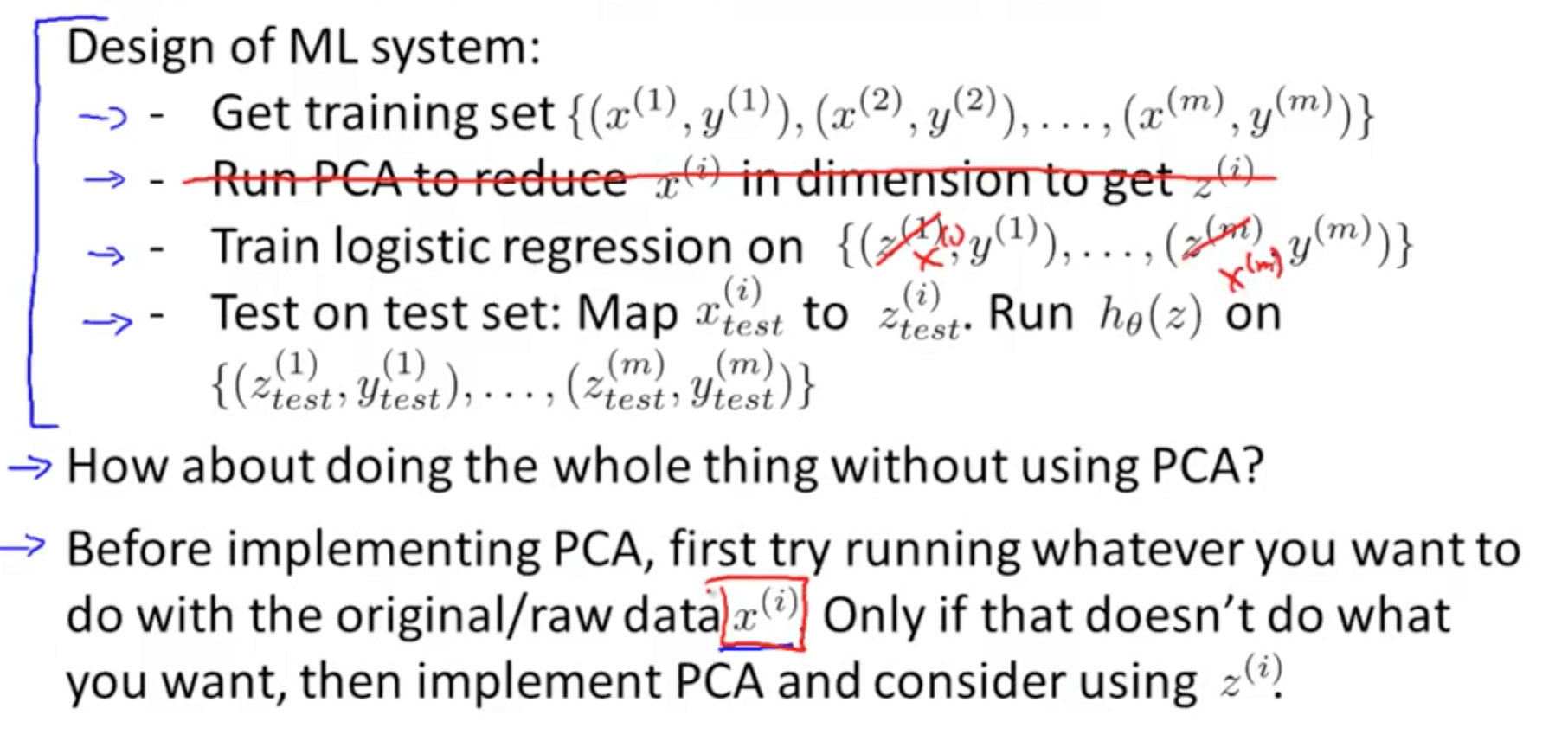Motivation of dimensionality reduction, Principal Component Analysis (PCA), and applying PCA.

## 1. Motivation

I would like to give full credits to the respective authors as these are my personal python notebooks taken from deep learning courses from Andrew Ng, Data School and Udemy :) This is a simple python notebook hosted generously through Github Pages that is on my main personal notes repository on https://github.com/ritchieng/ritchieng.github.io. They are meant for my personal review but I have open-source my repository of personal notes as a lot of people found it useful.

### 1a. Motivation I: Data Compression

• You are able to reduce the dimension of the data from 2D to 1D
• For example, pilot skill and pilot happiness can be reduced to pilot’s aptitude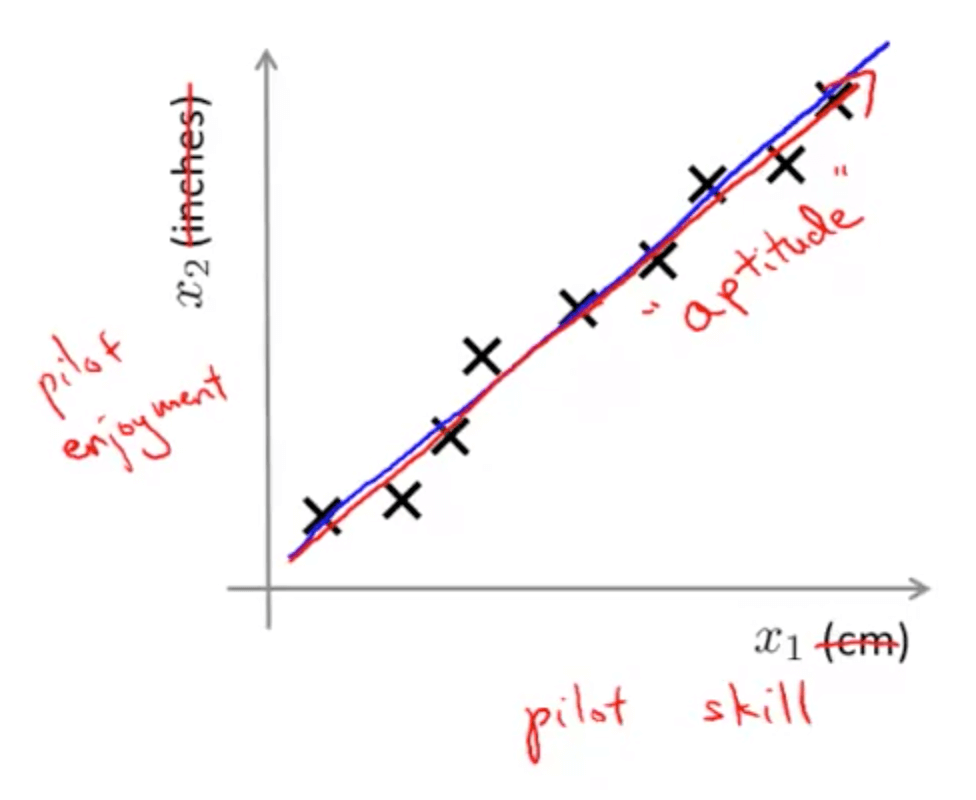• Generally, you can reduce x1 and x2 to z1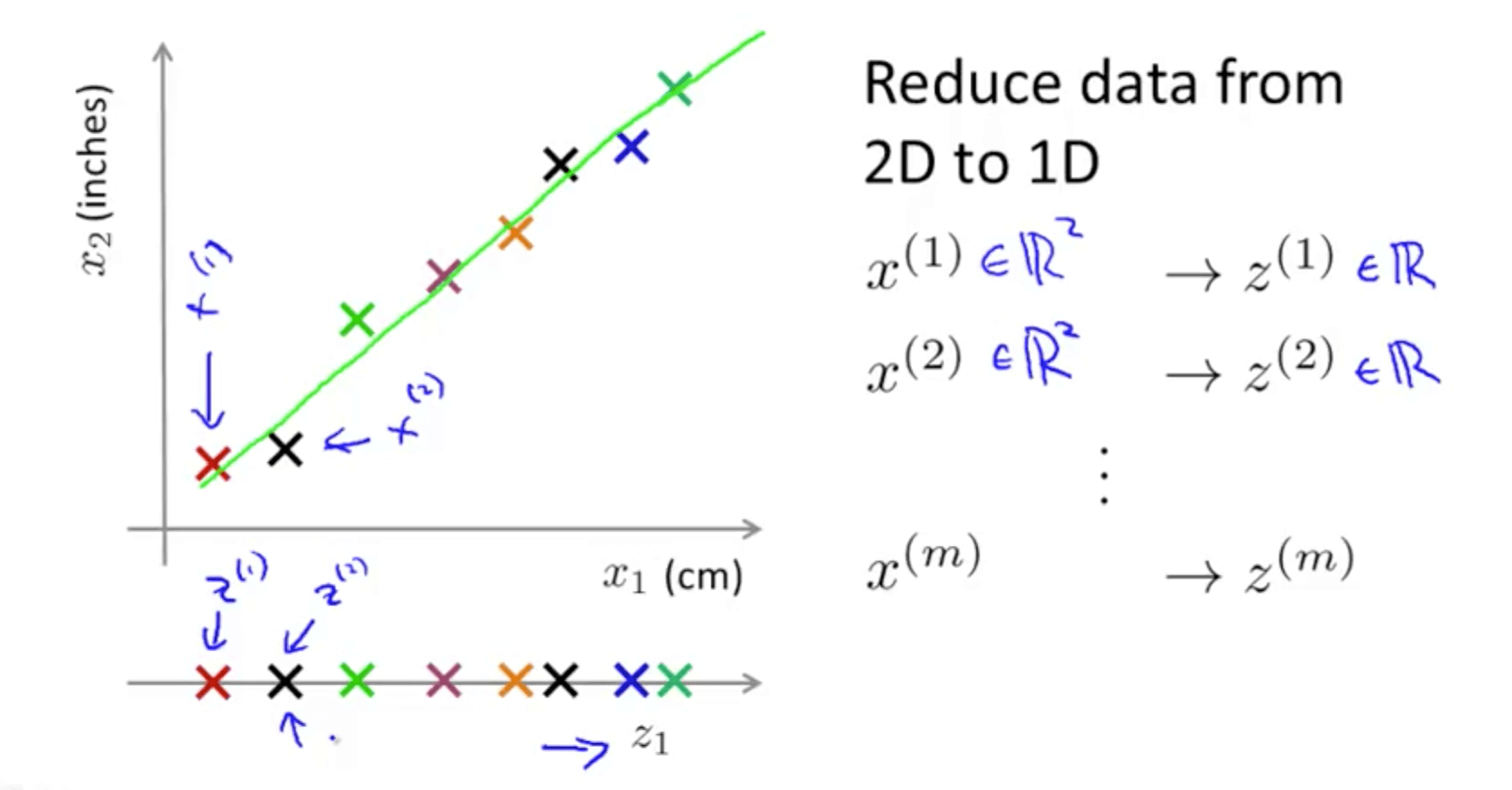• Your are able to reduce the dimension of the data from 3D to 2D
• Project the data such that they lie on a plane
• Specify two axes
• z1
• z2
• You would then be able to reduce the data’s dimension from 3D to 2D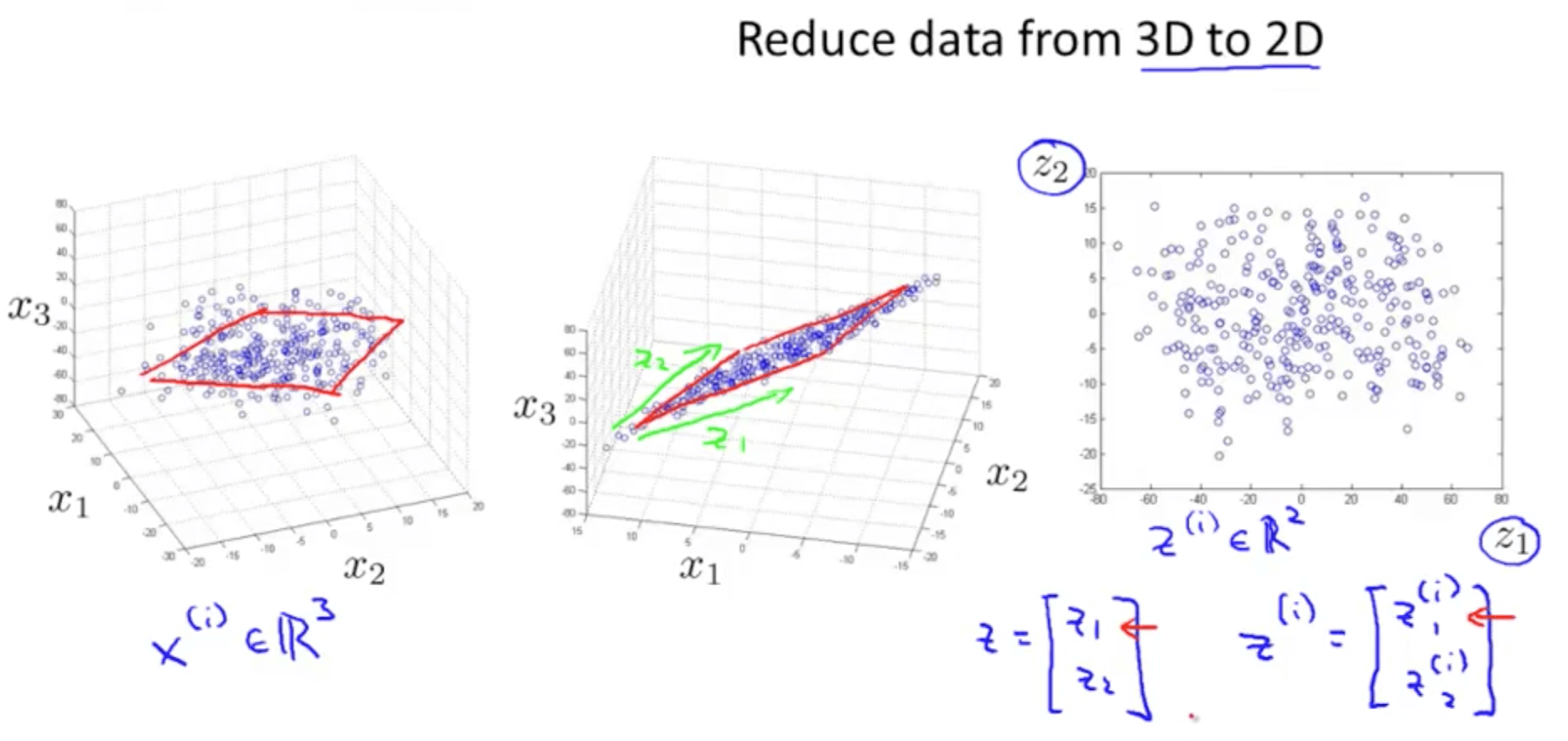### 1b. Motivation II: Visualization

• Given a set of data, how are able to examine the data such as this?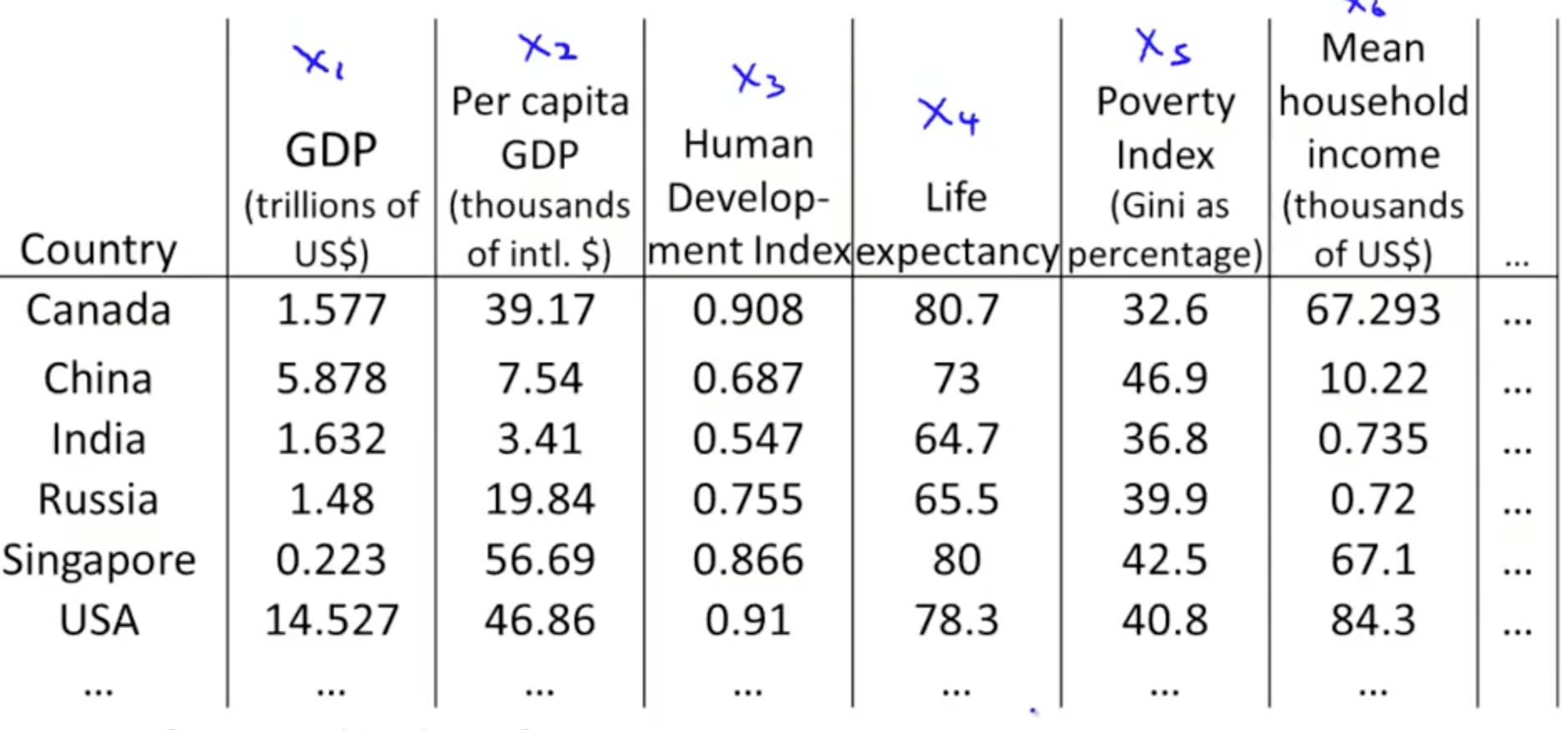• We can use reduce the data’s dimensionality from 50D to 2D
• Typically we do not know what the 2 dimensions’ meanings are
• But we can make sense of out of the 2 dimensions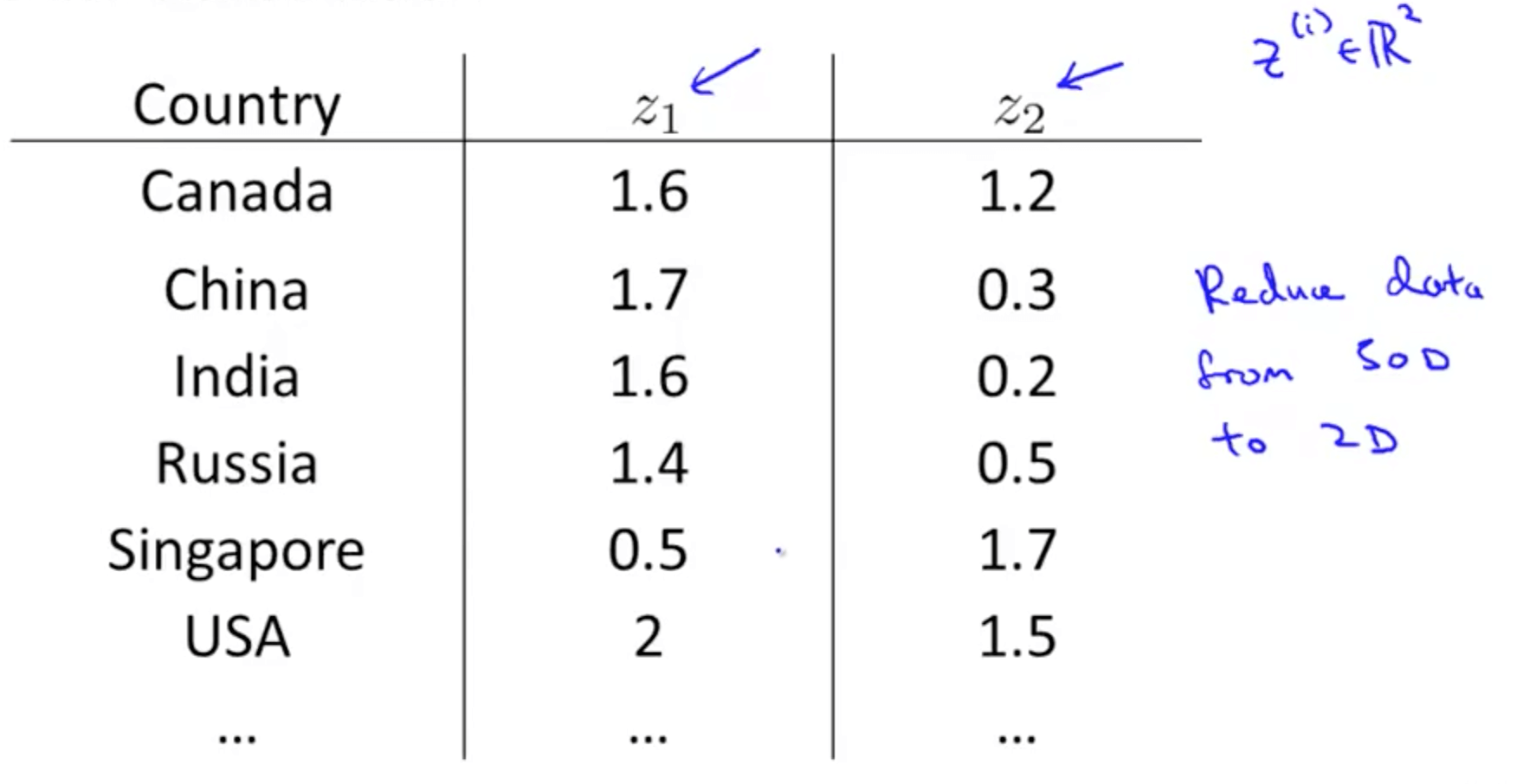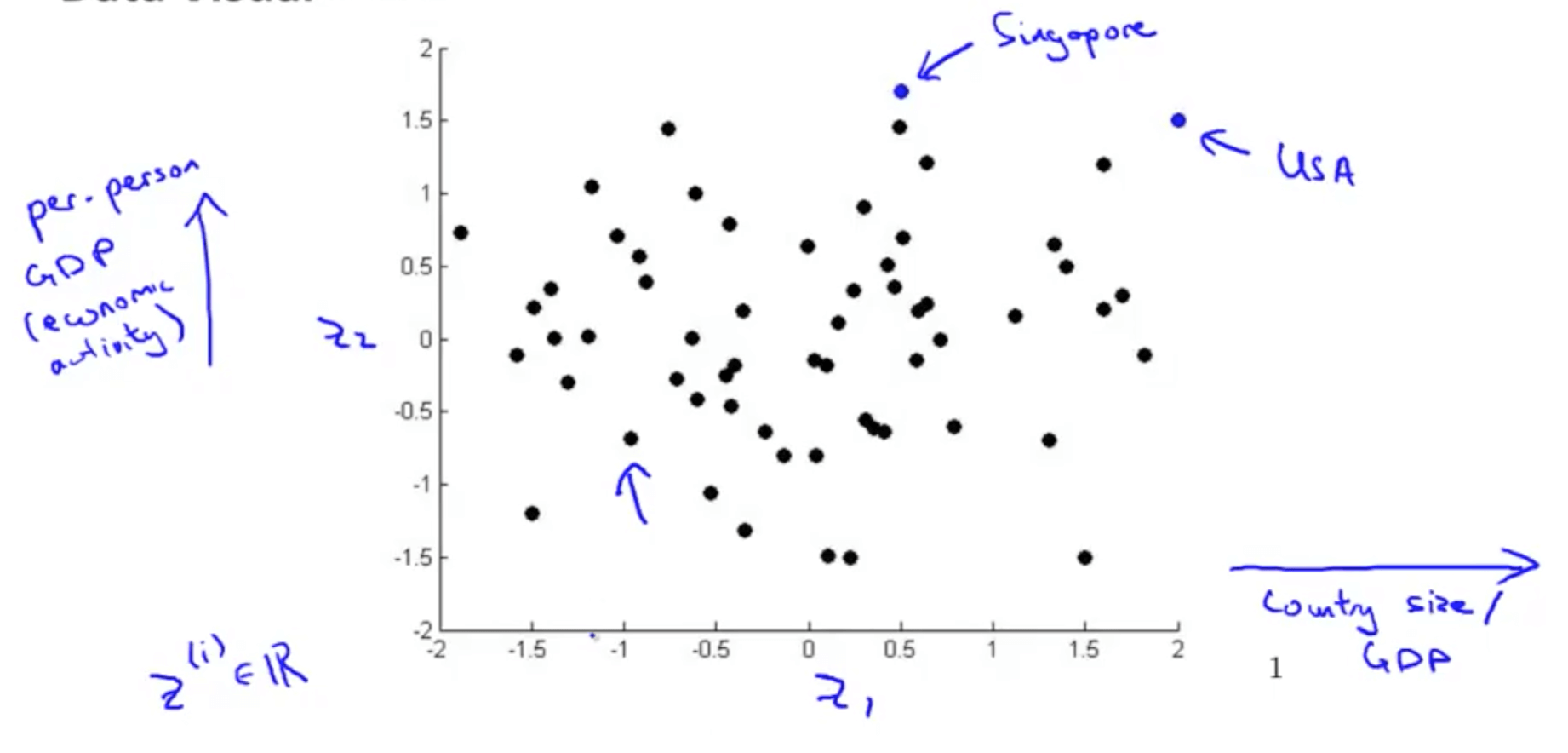## 2. Principal Component Analysis (PCA)

### 2a. PCA Problem Formation

• Let’s say we have the following 2D data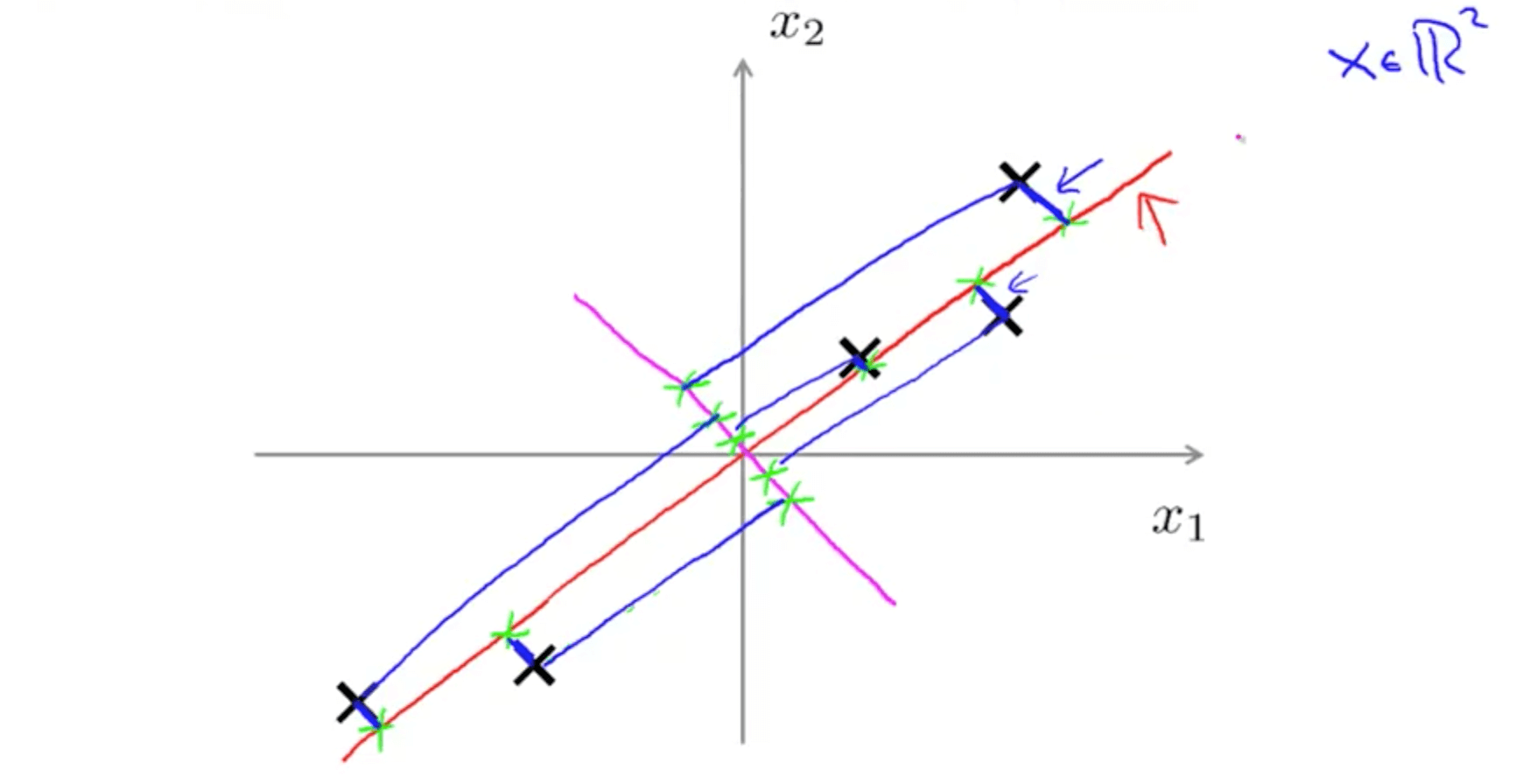1. We can project with a diagonal line (red line)
• PCA reduces the blue lines (the projection error)
• Before performing PCA, perform mean normalization (mean = 0) and feature scaling
2. We can also project with another diagonal line (magenta)
• But the projection errors are much larger
• Hence PCA would choose the red line instead of this magenta line
• Goal of PCA
• It’s trying to find a lower dimensional surface onto which to project the data, so as to minimize this squared projection error
• To minimize the square distance between each point and the location of where it gets projected.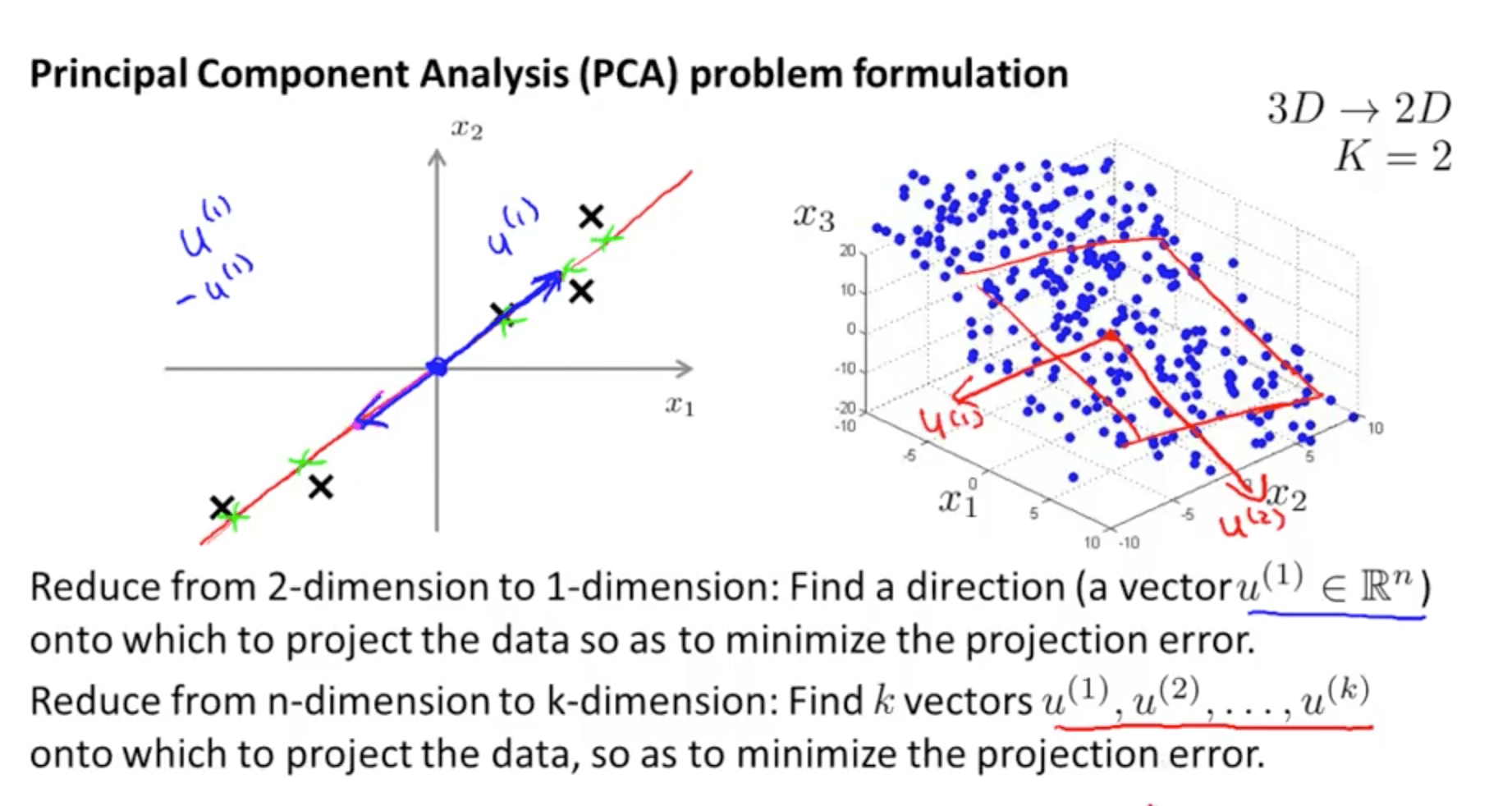• PCA is not linear regression
• PCA is a minimization of the orthogonal distance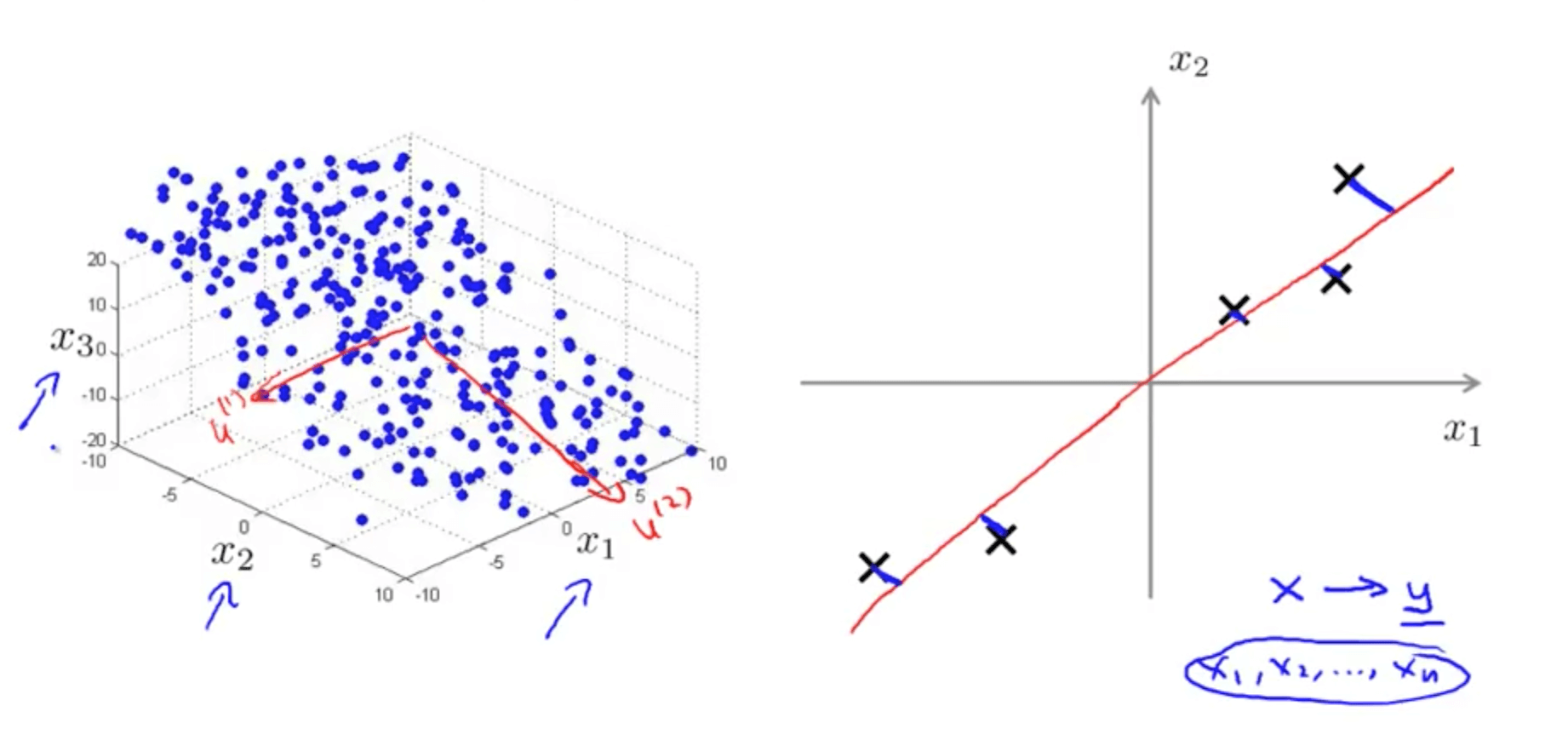### 2b. Principal Component Analysis Algorithm

• Data pre-processing step
• You must always do this before doing PCA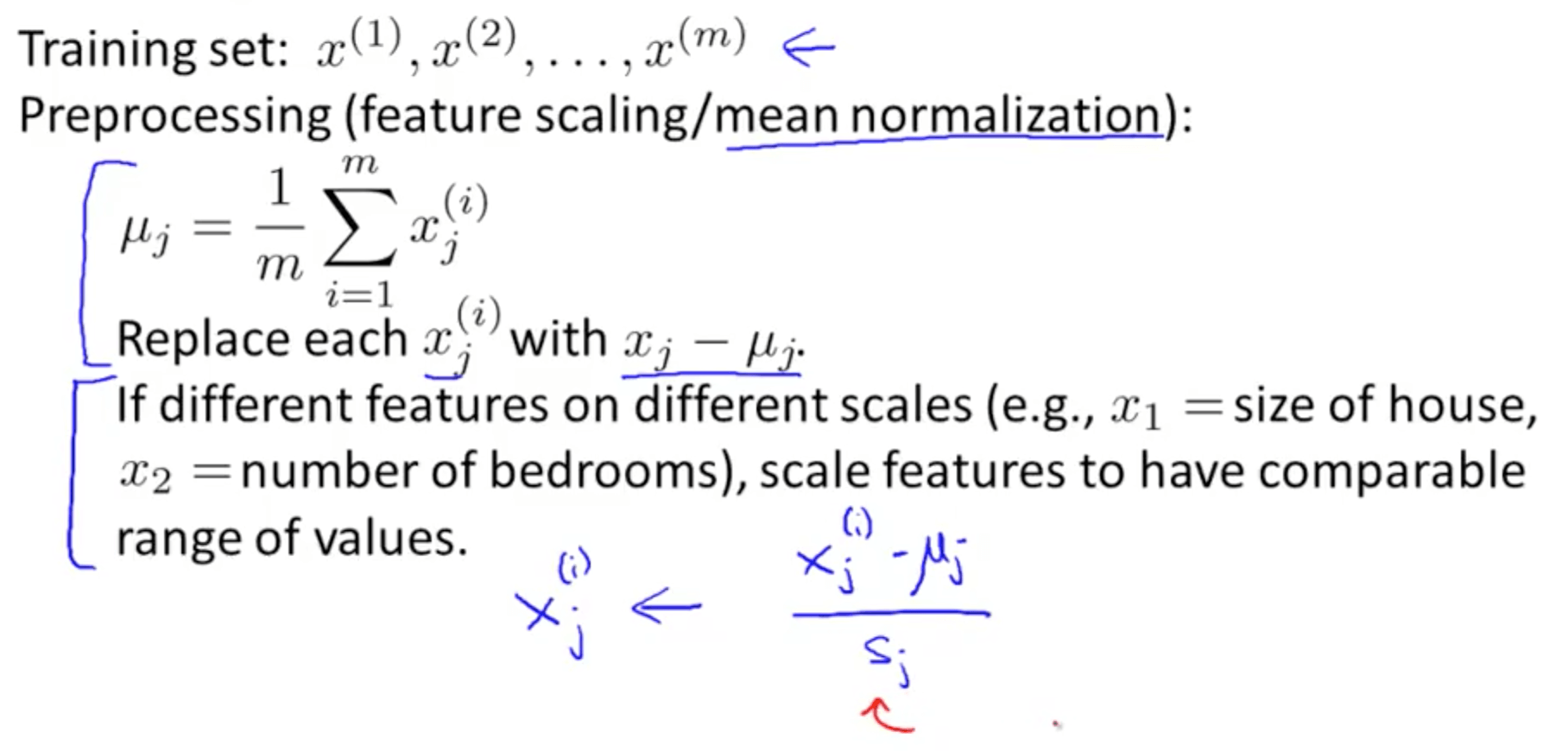• PCA intuition
• You need to compute the vector or vectors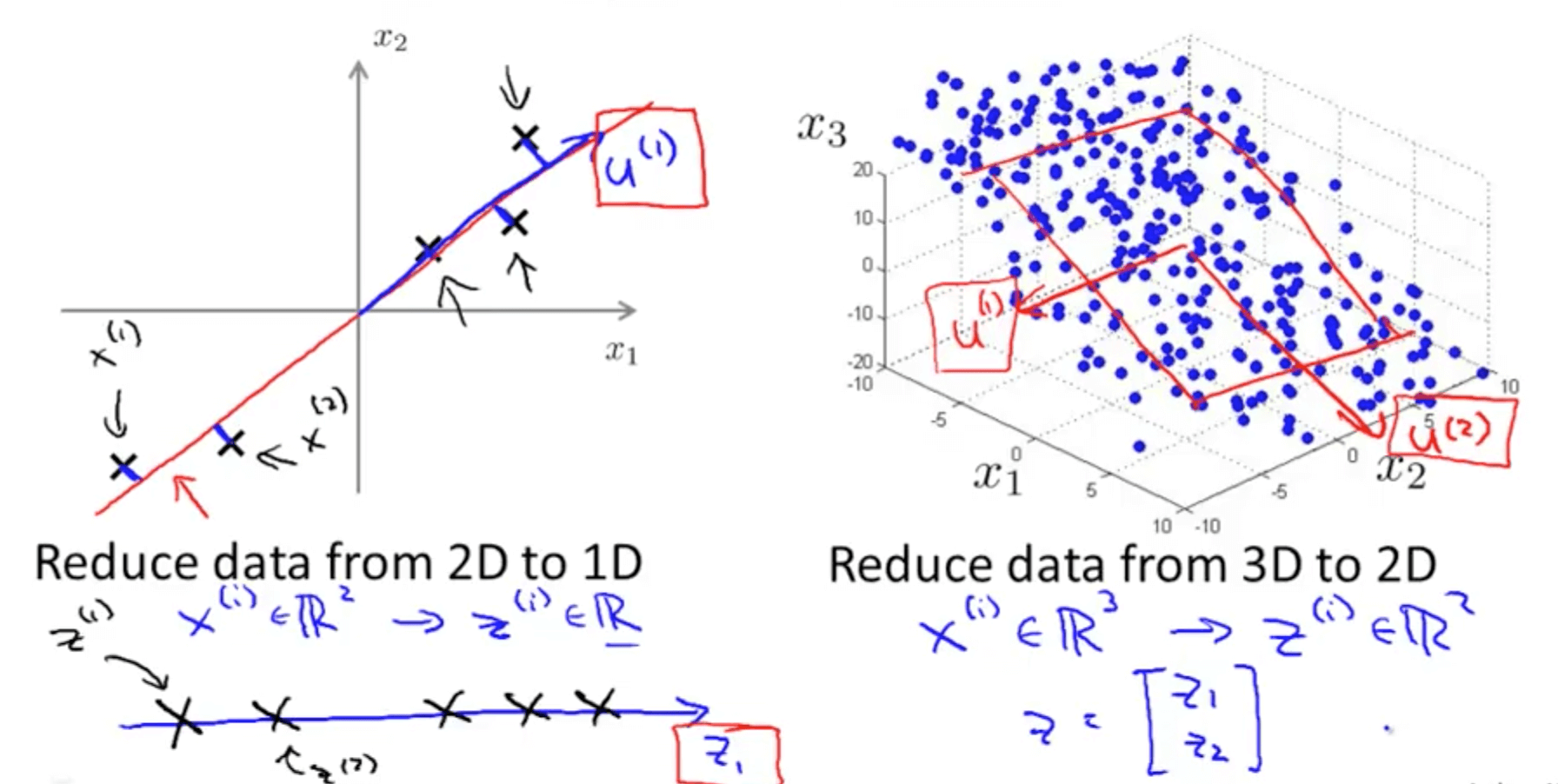• Left graph: compute vector z(1)
• Right graph: compute vector z(1) and z(2)
• Procedure
• You can use eig (eigen) or svd (singular value decomposition) but the later is more stable
• You can use any library in other languages that does singular value decomposition
• You will get 3 matrices: U, S and V
• But we only need matrix U where we manipulate to get z that is a k x 1 vector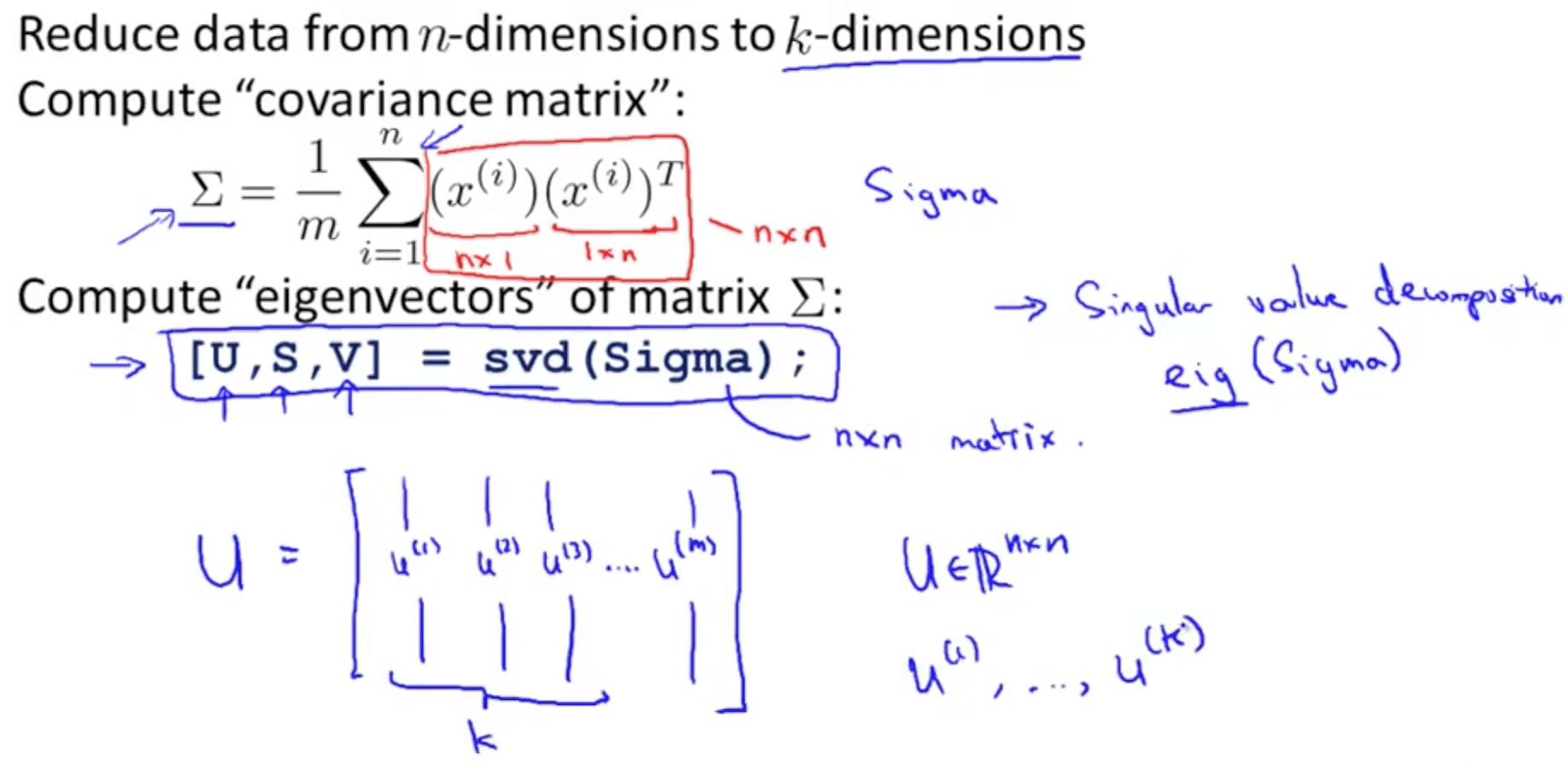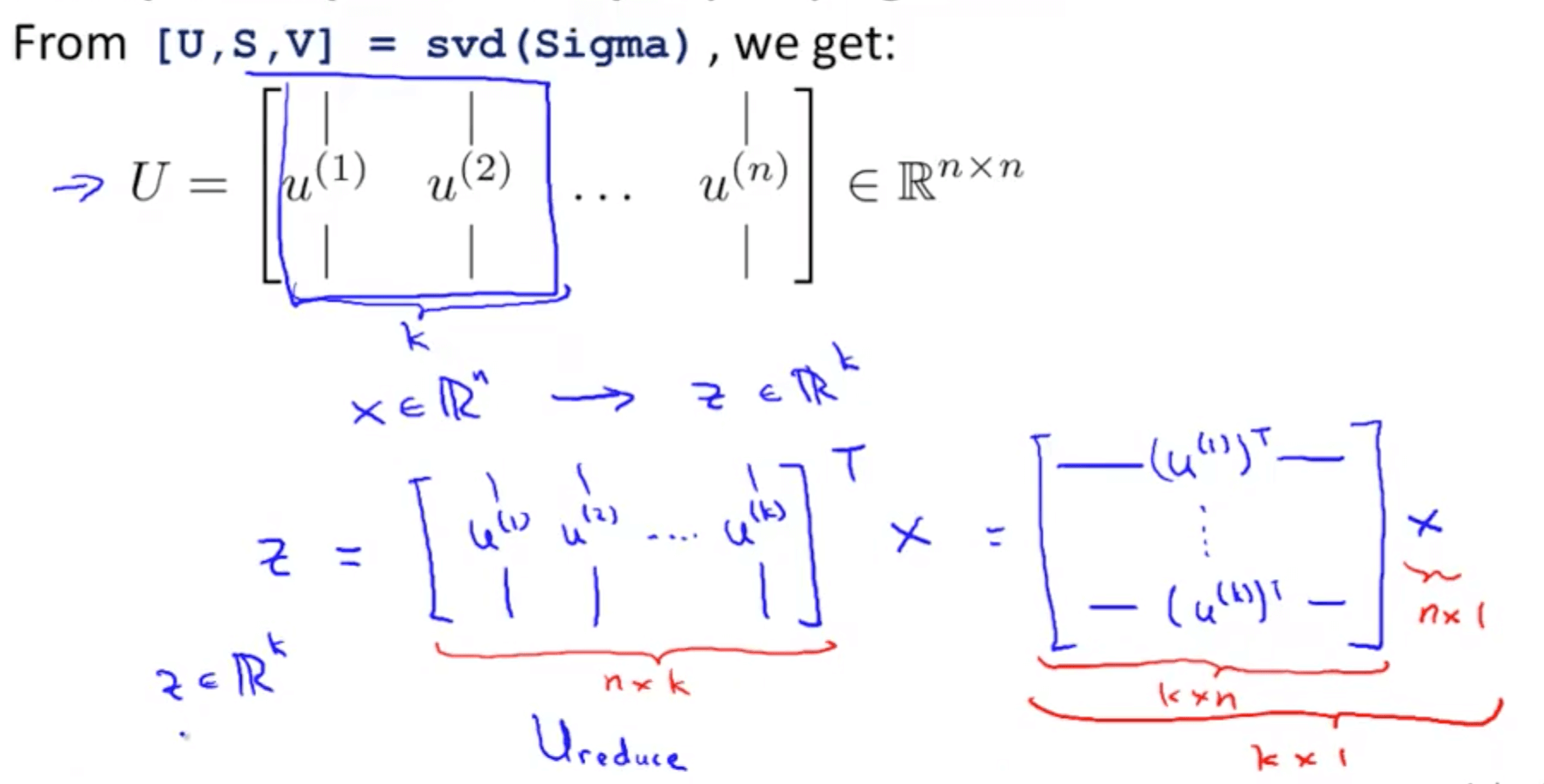• Summary of PCA algorithm in octave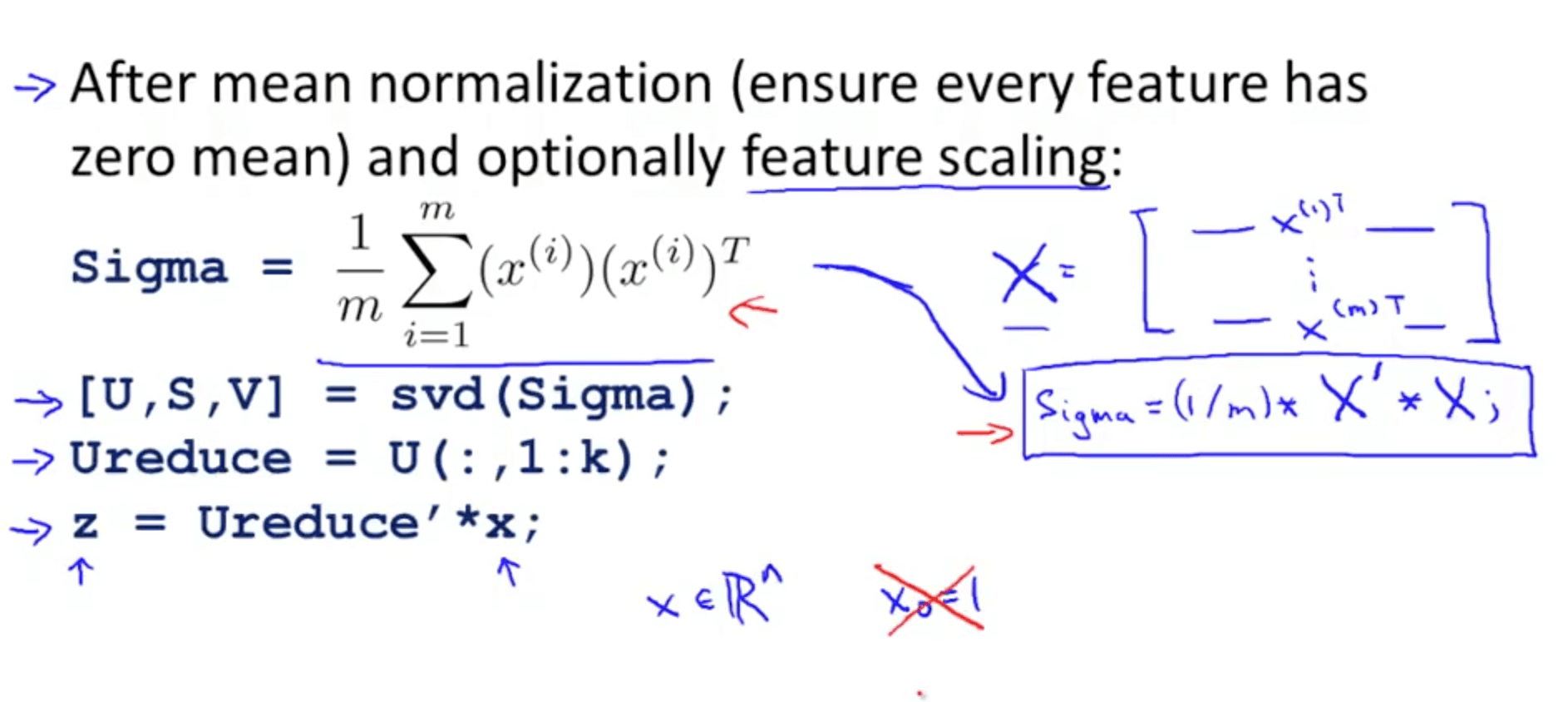## 3. Applying PCA

### 3a. Reconstruction from Compressed Representation

• We can go from lower dimensionality to higher dimensionality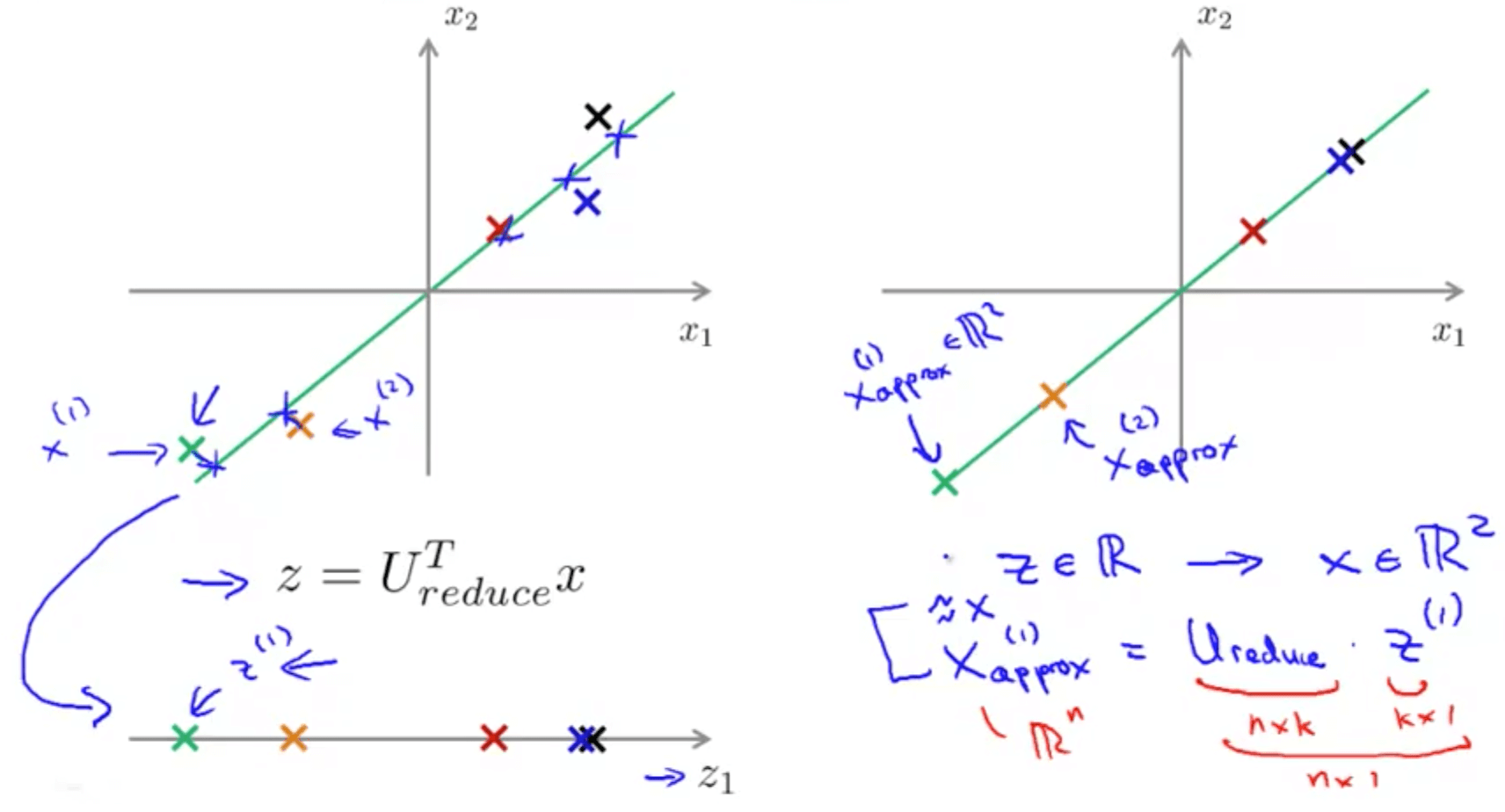### 3b. Choosing the Number of Principal Components

• k is the number of principal components
• But how do we choose k?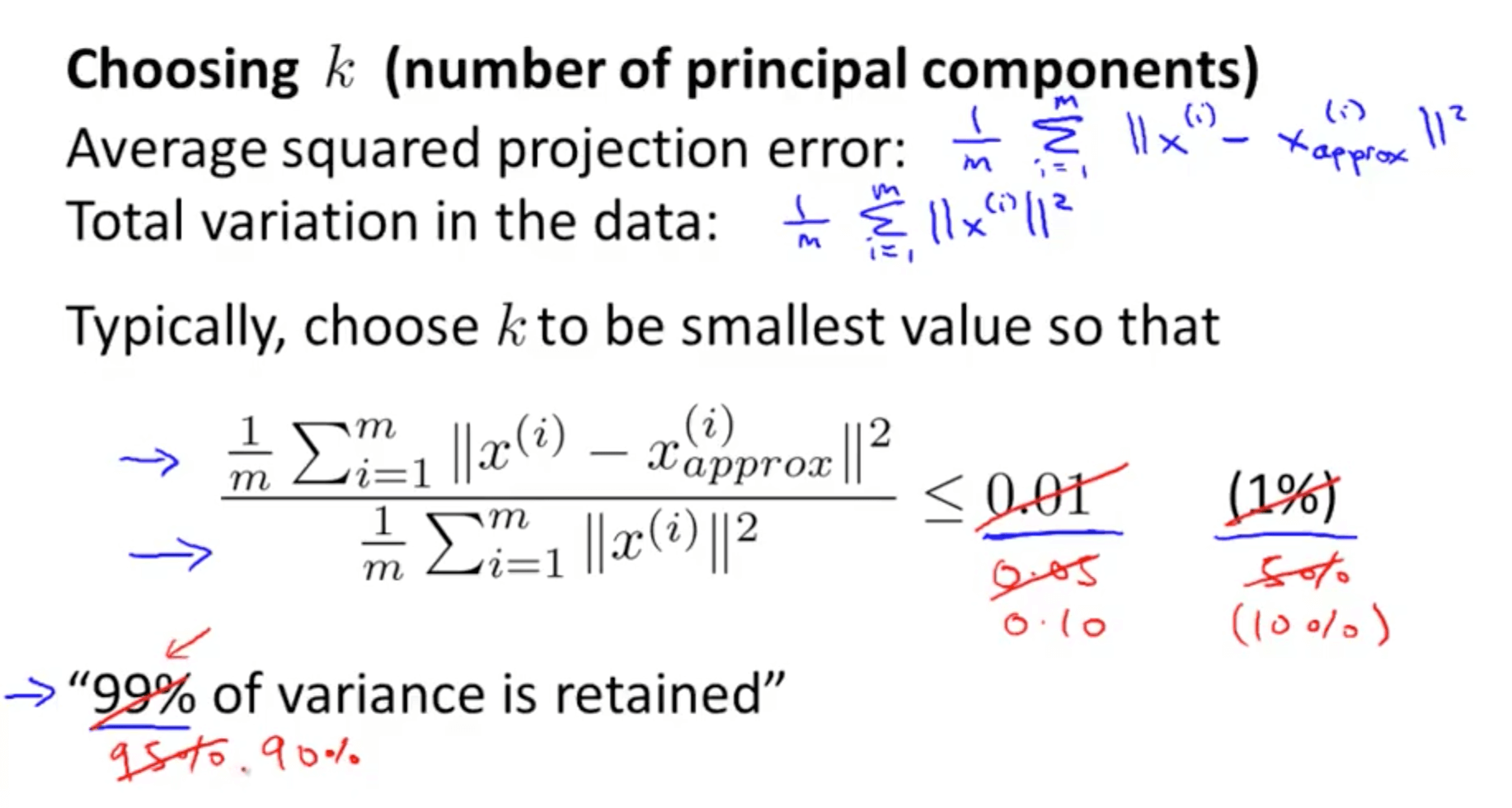• There is a more efficient method on the right compared to the left
• We then use the S matrix for calculations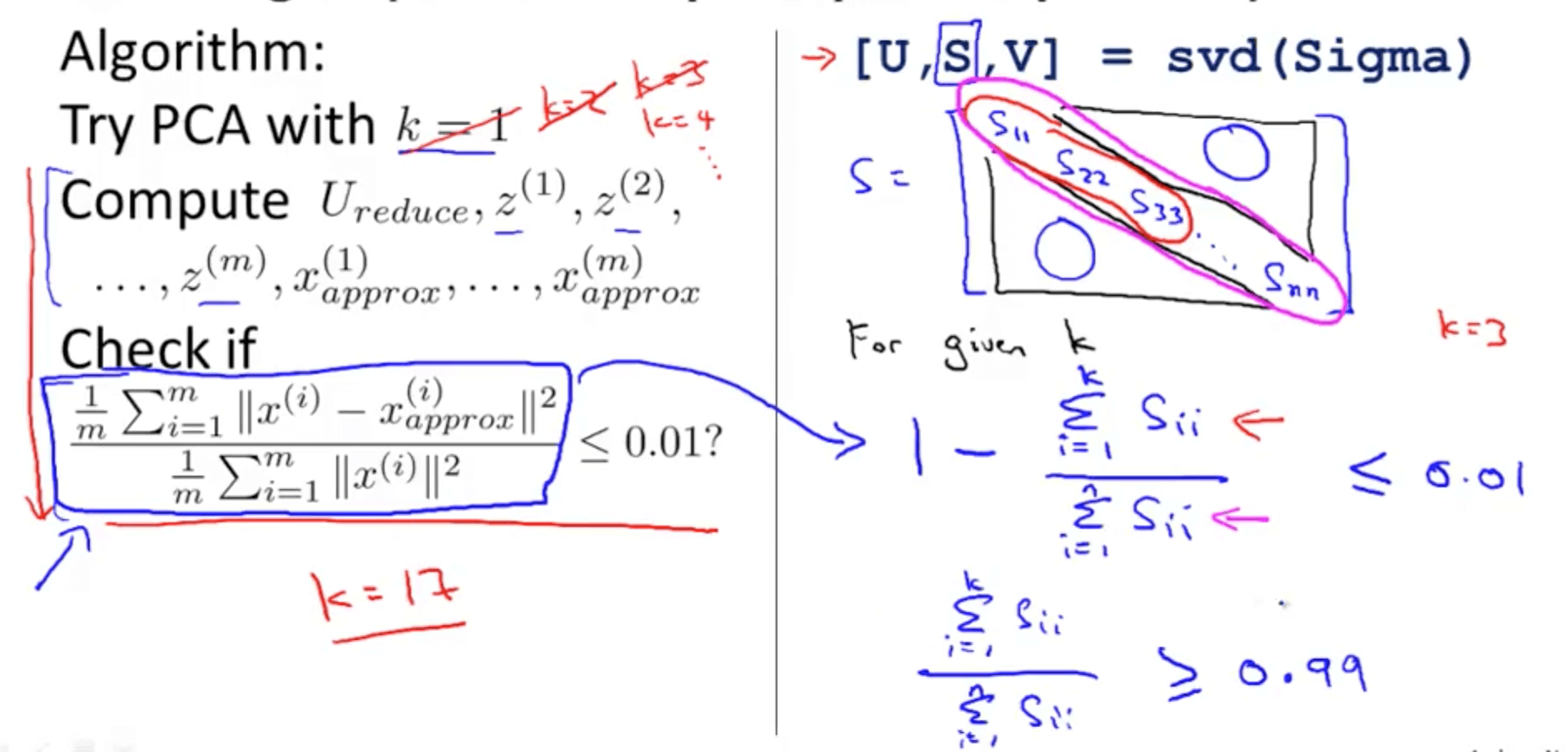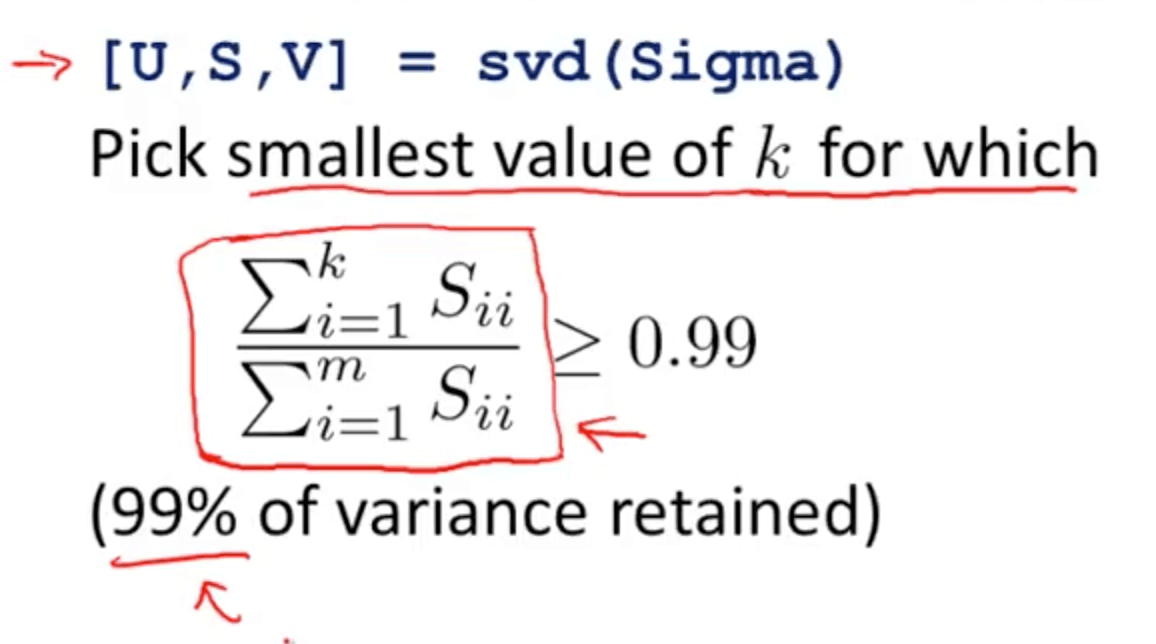• You would realise that PCA can retain a high percentage of the variance even after compressing the number of dimensions of the data

### 3c. Advice for Applying PCA

• Supervised learning
• For many data sets, we can reduce by 5-10x easily to ensure our learning algorithm runs much faster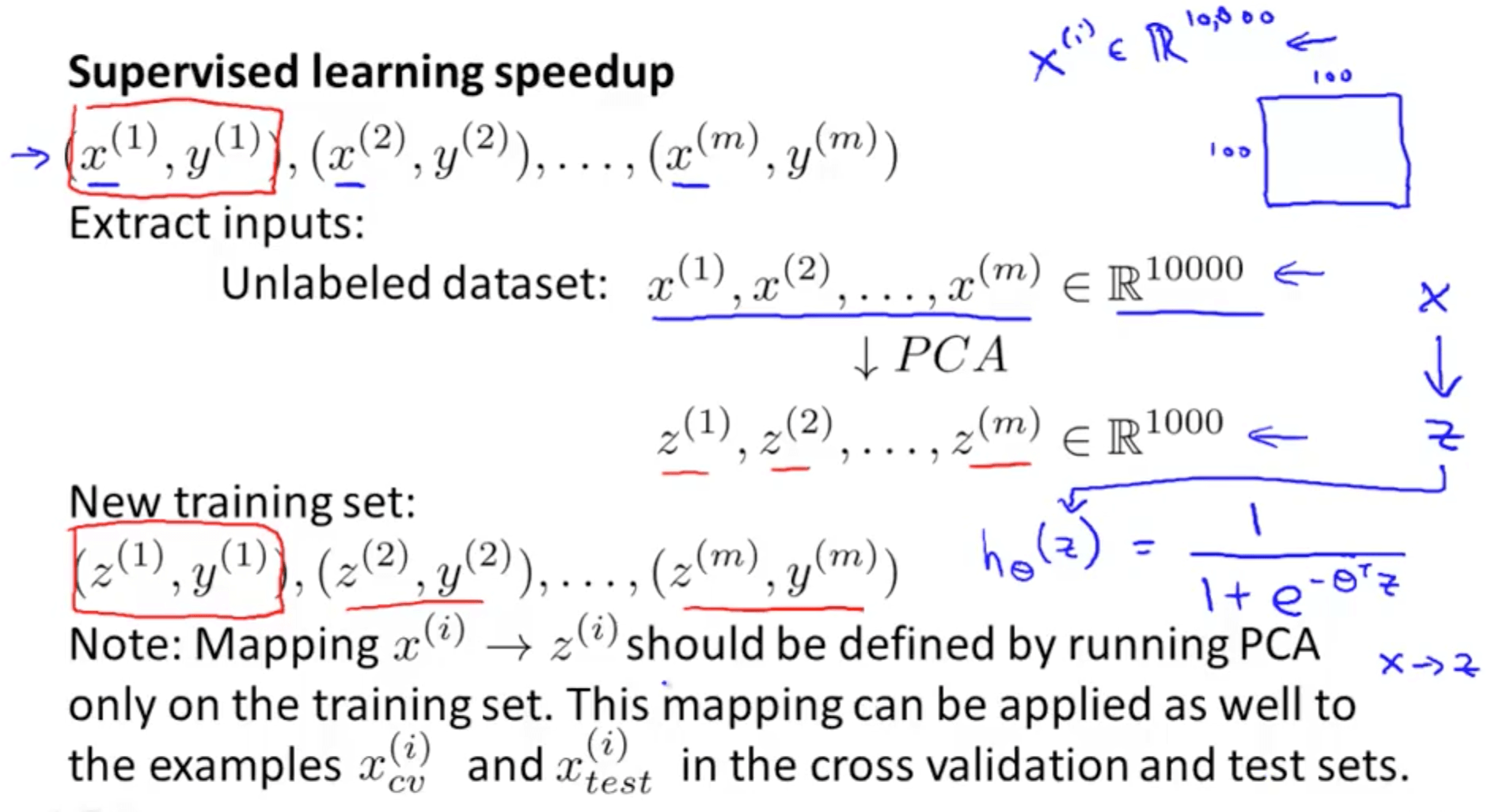• Application of PCA
1. Compression
• Reduce memory or disk needed to store data
• Speed up learning algorithm
• We choose k by percentage of variance retained
2. Visualization
• We choose only k = 2 or k = 3
• Regularization is better because it is less likely to throw away valuable information as it knows the labels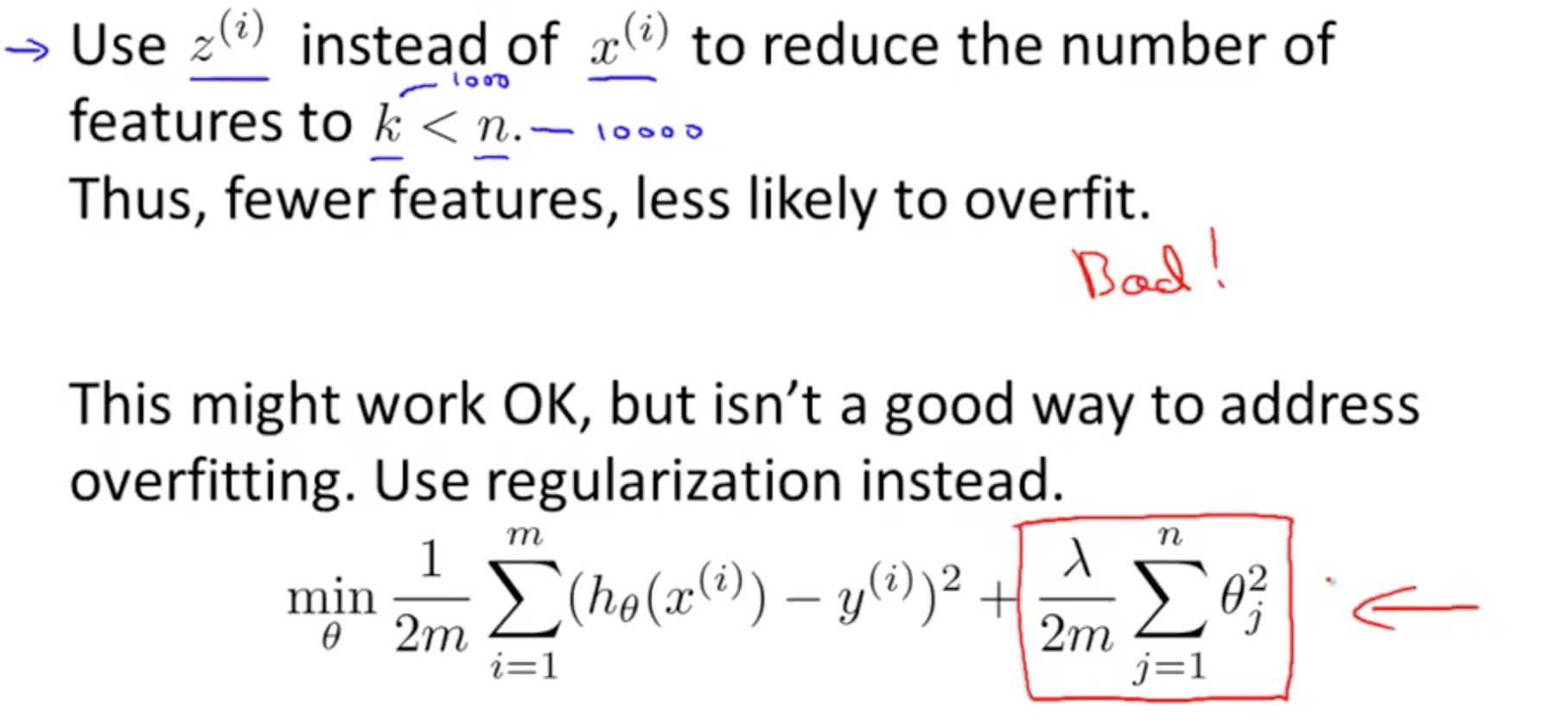2. Running PCA without consideration